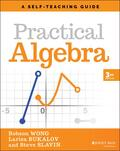John Wiley & Sons Practical AlgebraThe most practical, complete, and accessible guide for understanding algebra If you want to make se.. Product #: 978-1-119-71540-5 Regular price: \$21.40 \$21.40 Auf Lager

# Practical Algebra

## A Self-Teaching Guide

Wiley Self-Teaching Guides3. Auflage April 2022
512 Seiten, Softcover
Wiley & Sons Ltd

ISBN: 978-1-119-71540-5
John Wiley & Sons

Jetzt kaufen

### Preis: 22,90 €

Preis inkl. MwSt, zzgl. Versand

The most practical, complete, and accessible guide for understanding algebra

If you want to make sense of algebra, check out Practical Algebra: A Self-Teaching Guide. Written by two experienced classroom teachers, this Third Edition is completely revised to align with the Common Core Algebra I math standards used in many states. You'll get an overview of solving linear and quadratic equations, using ratios and proportions, decoding word problems, graphing and interpreting functions, modeling the real world with statistics, and other concepts found in today's algebra courses. This book also contains a brief review of pre-algebra topics, including arithmetic and fractions. It has concrete strategies that help diverse students to succeed, such as:
* over 500 images and tables that illustrate important concepts
* over 200 model examples with complete solutions
* almost 1,500 exercises with answers so you can monitor your progress

Practical Algebra emphasizes making connections to what you already know and what you'll learn in the future. You'll learn to see algebra as a logical and consistent system of ideas and see how it connects to other mathematical topics. This book makes math more accessible by treating it as a language. It has tips for pronouncing and using mathematical notation, a glossary of commonly used terms in algebra, and a glossary of symbols. Along the way, you'll discover how different cultures around the world over thousands of years developed many of the mathematical ideas we use today. Since students nowadays can use a variety of tools to handle complex modeling tasks, this book contains technology tips that apply no matter what device you're using. It also describes strategies for avoiding common mistakes that students make.

By working through Practical Algebra, you'll learn straightforward techniques for solving problems, and understand why these techniques work so you'll retain what you've learned. You (or your students) will come away with better scores on algebra tests and a greater confidence in your ability to do math.

Introduction

Chapter 1: Basic Concepts

Chapter 2: Fractions

Chapter 3: Linear Equations

Chapter 4: Ratios and Proportions

Chapter 5: Linear Inequalities

Chapter 6: Functions and Graphs with Two Variables

Chapter 7: Linear Functions and Their Graphs

Chapter 8: Operations with Polynomials

Chapter 10: Exponential Functions

Chapter 11: Sequences

Chapter 12: Summary of Functions

Chapter 13: Statistics

Formulas

Glossary of Mathematical Symbols

Glossary of Mathematical Terms

Acknowledgments